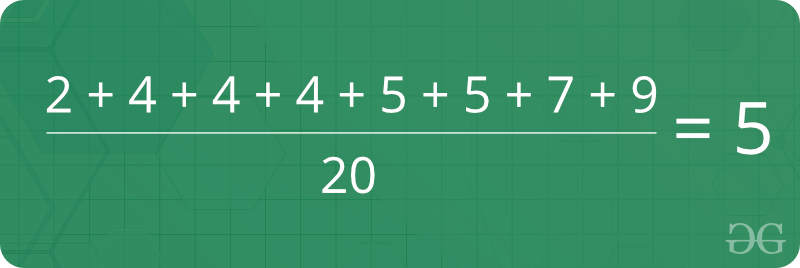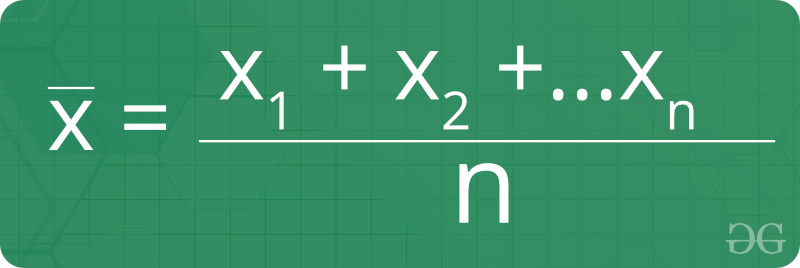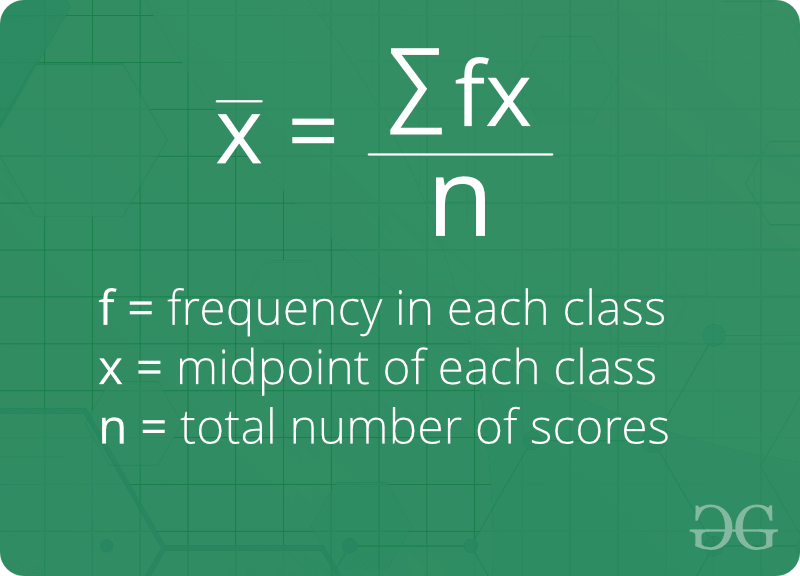Related Articles

# Mean

• Last Updated : 11 Dec, 2018

Mean is average of a given set of data. Let us consider below example 2, 4, 4, 4, 5, 5, 7, 9 the mean (average) of a given set of data is 51. The mean (or average) is the most popular and well known measure of central tendency.
2. It can be used with both discrete and continuous data, although its use is most often with continuous data.
3. There are other types of means.Geometric mean, Harmonic mean and Arithmetic mean.
4. Mean is the only measure of central tendency where the sum of the deviations of each value from the mean is always zero.

Formula of mean of ungrouped data :Formula of mean of grouped data :How to find Mean ?
Given n size unsorted array, find its mean.

Mean of an array = (sum of all elements) / (number of elements)

Since the array is not sorted here, we sort the array first, then apply above formula.

Examples:

```Input  : {1, 3, 4, 2, 6, 5, 8, 7}
Output : Mean = 4.5

Sum of the elements is 1 + 3 + 4 + 2 + 6 +
5 + 8 + 7 = 36
Mean = 36/8 = 4.5

Input  : {4, 4, 4, 4, 4}
Output : Mean = 4```

Below is the code implementation:

## C++

 `// CPP program to find mean``#include ``using` `namespace` `std;`` ` `// Function for calculating mean``double` `findMean(``int` `a[], ``int` `n)``{``    ``int` `sum = 0;``    ``for` `(``int` `i = 0; i < n; i++)``        ``sum += a[i];`` ` `    ``return` `(``double``)sum / (``double``)n;``}`` ` `// Driver program``int` `main()``{``    ``int` `a[] = { 1, 3, 4, 2, 7, 5, 8, 6 };``    ``int` `n = ``sizeof``(a) / ``sizeof``(a);``    ``cout << ``"Mean = "` `<< findMean(a, n) << endl;``    ``return` `0;``}`

## Java

 `// Java program to find mean``import` `java.util.*;`` ` `class` `GFG {`` ` `    ``// Function for calculating mean``    ``public` `static` `double` `findMean(``int` `a[], ``int` `n)``    ``{``        ``int` `sum = ``0``;``        ``for` `(``int` `i = ``0``; i < n; i++)``            ``sum += a[i];`` ` `        ``return` `(``double``)sum / (``double``)n;``    ``}`` ` `    ``// Driver program``    ``public` `static` `void` `main(String args[])``    ``{``        ``int` `a[] = { ``1``, ``3``, ``4``, ``2``, ``7``, ``5``, ``8``, ``6` `};``        ``int` `n = a.length;``        ``System.out.println(``"Mean = "` `+ findMean(a, n));``    ``}``}`

## Python3

 `# Python3 program to find mean `` ` `# Function for calculating mean``def` `findMean(a, n):`` ` `    ``sum` `=` `0``    ``for` `i ``in` `range``( ``0``, n):``        ``sum` `+``=` `a[i]``     ` `    ``return` `float``(``sum` `/` `n)`` ` `# Driver program``a ``=` `[ ``1``, ``3``, ``4``, ``2``, ``7``, ``5``, ``8``, ``6` `]``n ``=` `len``(a)``print``(``"Mean ="``, findMean(a, n))`

## C#

 `// C# program to find mean``using` `System;`` ` `class` `GFG {``    ``// Function for``    ``// calculating mean``    ``public` `static` `double` `findMean(``int``[] a, ``int` `n)``    ``{``        ``int` `sum = 0;``        ``for` `(``int` `i = 0; i < n; i++)``            ``sum += a[i];`` ` `        ``return` `(``double``)sum / (``double``)n;``    ``}`` ` `    ``// Driver Code``    ``public` `static` `void` `Main()``    ``{``        ``int``[] a = { 1, 3, 4, 2,``                    ``7, 5, 8, 6 };``        ``int` `n = a.Length;``        ``Console.Write(``"Mean = "` `+ findMean(a, n) + ``"\n"``);``    ``}``}`

## PHP

 ``

Output:
`Mean = 4.5`

Time Complexity to find mean = O(n)

Basic Program related to Mean

Recent Articles on Mean!

Attention reader! Don’t stop learning now. Get hold of all the important mathematical concepts for competitive programming with the Essential Maths for CP Course at a student-friendly price. To complete your preparation from learning a language to DS Algo and many more,  please refer Complete Interview Preparation Course.

My Personal Notes arrow_drop_up